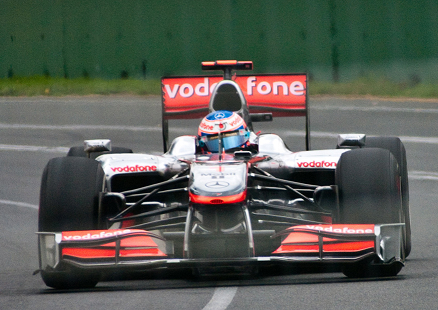$$\require{cancel}$$

# 6.1: Prelude to Uniform Circular Motion and Gravitation

•• Contributed by OpenStax
• General Physics at OpenStax CNX

Many motions, such as the arc of a bird’s flight or Earth’s path around the Sun, are curved. Recall that Newton’s first law tells us that motion is along a straight line at constant speed unless there is a net external force. We will therefore study not only motion along curves, but also the forces that cause it, including gravitational forces. In some ways, this chapter is a continuation of Dynamics: Newton's Laws of Motion as we study more applications of Newton’s laws of motion.Figure $$\PageIndex{1}$$: This Australian Grand Prix Formula 1 race car moves in a circular path as it makes the turn. Its wheels also spin rapidly—the latter completing many revolutions, the former only part of one (a circular arc). The same physical principles are involved in each. (credit: Richard Munckton)

This chapter deals with the simplest form of curved motion, uniform circular motion, motion in a circular path at constant speed. Studying this topic illustrates most concepts associated with rotational motion and leads to the study of many new topics we group under the name rotation. Pure rotational motion occurs when points in an object move in circular paths centered on one point. Pure translational motion is motion with no rotation. Some motion combines both types, such as a rotating hockey puck moving along ice.

# Glossary

uniform circular motion
the motion of an object in a circular path at constant speed

# Contributors

Paul Peter Urone (Professor Emeritus at California State University, Sacramento) and Roger Hinrichs (State University of New York, College at Oswego) with Contributing Authors: Kim Dirks (University of Auckland) and Manjula Sharma (University of Sydney). This work is licensed by OpenStax University Physics under a Creative Commons Attribution License (by 4.0).